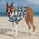1424 lượt xem
1424
This is a very simple idea - an average of RSI and the Stochastic Oscillator. However it offers plenty of flexibility for tuning to your requirements. You can change the lengths of either indicator and the weighting of each. By default it's set to 50/50 (just change the percent RSI to adjust). There is also an optional EMA which can be used as a signal line.
This idea comes from Greg Morris who likes to use it for trend following. I would buy when the indicator hits the overbought line and sell when it goes below 50.
//Scripted by Blind Freddy
//See my blog at http://blindfreddy.postagon.com for learning and picks
//Hybrid RSI-Stochastic Indicator, with optional EMA line
study(title="RSI-Stoch Hybrid", shorttitle="RSI-Stoch", overlay=false)
len = input(14, minval=1, title="RSI Length")
lenstoch = input(26, minval=1, title="Stochastic Length")
thepercent = input(50, minval=0, maxval = 100, title="Percent RSI")
len2 = input(5, minval=1, title="EMA (Signal) Length")
thersi= rsi(close,len)
thestoch=stoch(close,high,low,lenstoch)
thehybrid=thersi*thepercent/100 + thestoch*(100-thepercent)/100
theEMA = ema(thehybrid,len2)
plot(thehybrid, title="RSI-Stoch Hybrid", style=line, linewidth=2, color=teal)
plot(theEMA, title="EMA", style=line, linewidth=2, color=gray)
band2 = hline(80, title="Upper Line", linestyle=dashed, linewidth=1)
band1 = hline(50, title="Upper Line", linestyle=dashed, linewidth=1)
band0 = hline(20, title="Lower Line", linestyle=dashed, linewidth=1)
fill(band2, band0, color=blue, transp=90)blindfreddy
..with exponential smoothing of the stochastic and RSI as an option before combining them
Phản hồi